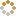﻿ Ningbo University of Technology Online Judge ::  D Minimums of Sum
• ###  D Minimums of Sum

• 时间限制: 1000 ms　内存限制: 65535 K
• 问题描述
• When Zero learned Wodex was enlisted soldiers, he led the army launch a surprise attack, so Wodex with no experience had a fiasco.

Wodex ran away with his three partners. They dicided to compete with Zero only by own. So, they came to a castle which belonged to Zero.
When they came in the castle, there were a locked door in front of them. And there were d * d lattices, each lattice had a integer on it.
At the same time, horn sounded:
If you can find d minimum integers, you can open the door. You should choose only one number at each line and the sum of d numbers will be one minimum, so you should repeat d times to get d numbers of minimums of sum.
You can not choose the same position of all lattices likes before you choosed, changing at least one.
Can you help him to find these d sums?

• 输入
• There will be several test cases. The first line of each case contains an integer d (1 < d < 450). Each of the following d lines contains d positive integers in each line. Each of these integers does not exceed 10,000 and will be more than 0.
• 输出
• For each test, d integers output from small to large.
• 样例输入
• ```3
1 8 5
9 2 5
10 7 6
2
1 1
1 2```
• 样例输出
• ```9 10 12
2 2```
• 提示
• ```At first case, 9 = 1 + 2 + 6; 10 = 1 + 2 + 7; 12 = 1 + 5 + 6.
At second case, 2 = 1 + 1; 2 = 1 + 1. ```
• 来源
• `Hungar`
• 操作
﻿